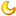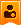please choosego to mobile | Continue to access the PC version

# About OrangePI PC CVBS again

Registered membercredits
69Published in 2016-11-19 12:27:47 | Show all floors |Read mode
 this time i buy the Multimeter and you guess what? According to the OrangePI PC design plan 3.5mm jack is < Right | Left | CVBS | GND but now: < CVBS | Right | Left | GND then i try to Change plug position it's works(but without video signal just a black...) and according to wiki (from here http://linux-sunxi.org/Display#Using_two_displays ) i think will be add this thing? [tv_out_dac_para] dac_used = 1 dac0_src = 4 dac1_src = 5 dac2_src = 6 dac3_src = 0Copy codebut not! this time even black screen signal is gone so i check the bin(using bin2fex see the second post) there's the code is that wiki wrong?!

Registered membercredits
69Author| Published in 2016-11-19 12:28:51 | Show all floors
 this is code(part1) [product] version = "100" machine = "orangepi-plus" [platform] debug_mode = 1 eraseflag = 1 next_work = 2 [target] boot_clock = 1536 storage_type = -1 [key_detect_en] keyen_flag = 0 [fel_key] fel_key_max = 7 fel_key_min = 2 [card_boot] logical_start = 40960 sprite_work_delay = 500 sprite_err_delay = 200 sprite_gpio0 = port:PA15<1> next_work = 3 [box_start_os] used = 1 start_type = 1 irkey_used = 1 pmukey_used = 1 pmukey_num = 3 led_power = 0 led_state = 0 [boot_init_gpio] used = 1 gpio0 = port:PA15<1><1> gpio1 = port:PG11<1><1> [recovery_para] used = 1 mode = 2 recovery_key = port:PL04<0> [pm_para] standby_mode = 1 [card0_boot_para] card_ctrl = 0 card_high_speed = 1 card_line = 4 sdc_d1 = port:PF00<2><1><2> sdc_d0 = port:PF01<2><1><2> sdc_clk = port:PF02<2><1><2> sdc_cmd = port:PF03<2><1><2> sdc_d3 = port:PF04<2><1><2> sdc_d2 = port:PF05<2><1><2> [card2_boot_para] card_ctrl = 2 card_high_speed = 1 card_line = 8 sdc_cmd = port:PC06<3><1><2> sdc_clk = port:PC05<3><1><2> sdc_d0 = port:PC08<3><1><2> sdc_d1 = port:PC09<3><1><2> sdc_d2 = port:PC10<3><1><2> sdc_d3 = port:PC11<3><1><2> sdc_d4 = port:PC12<3><1><2> sdc_d5 = port:PC13<3><1><2> sdc_d6 = port:PC14<3><1><2> sdc_d7 = port:PC15<3><1><2> sdc_2xmode = 1 sdc_ddrmode = 1 [twi_para] twi_port = 0 twi_scl = port:PA11<2> twi_sda = port:PA12<2> [uart_para] uart_debug_port = 0 uart_debug_tx = port:PA04<2><1> uart_debug_rx = port:PA05<2><1> [force_uart_para] force_uart_port = 0 force_uart_tx = port:PF02<3><1> force_uart_rx = port:PF04<3><1> [jtag_para] jtag_enable = 0 jtag_ms = port:PA00<3> jtag_ck = port:PA01<3> jtag_do = port:PA02<3> jtag_di = port:PA03<3> [clock] pll_video = 297 pll_ve = 402 pll_periph0 = 600 pll_gpu = 576 pll_periph1 = 600 pll_de = 864 [dram_para] dram_clk = 672 dram_type = 3 dram_zq = 0x3b3bfb dram_odt_en = 1 dram_para1 = 283377664 dram_para2 = 0 dram_mr0 = 6208 dram_mr1 = 64 dram_mr2 = 24 dram_mr3 = 2 dram_tpr0 = 0x48a192 dram_tpr1 = 0x1c2418d dram_tpr2 = 0x76051 dram_tpr3 = 0x0 dram_tpr4 = 0x0 dram_tpr5 = 0x0 dram_tpr6 = 0x64 dram_tpr7 = 0x0 dram_tpr8 = 0x0 dram_tpr9 = 0x0 dram_tpr10 = 0x0 dram_tpr11 = 0x6aaa0000 dram_tpr12 = 0x7979 dram_tpr13 = 0x800800 [wakeup_src_para] cpu_en = 0 cpu_freq = 48 pll_ratio = 273 dram_selfresh_en = 1 dram_freq = 36 wakeup_src0 = wakeup_src_wl = port:PG10<4><0> [twi0] twi_used = 1 twi_scl = port:PA11<2> twi_sda = port:PA12<2> [twi1] twi_used = 1 twi_scl = port:PA18<3> twi_sda = port:PA19<3> [twi2] twi_used = 0 twi_scl = port:PE12<3> twi_sda = port:PE13<3> [uart0] uart_used = 1 uart_port = 0 uart_type = 2 uart_tx = port:PA04<2><1> uart_rx = port:PA05<2><1> [uart1] uart_used = 0 uart_port = 1 uart_type = 2 uart_tx = port:PG06<2><1> uart_rx = port:PG07<2><1> uart_rts = port:PG08<2><1> uart_cts = port:PG09<2><1> [uart2] uart_used = 1 uart_port = 2 uart_type = 4 uart_tx = port:PA00<2><1> uart_rx = port:PA01<2><1> uart_rts = port:PA02<2><1> uart_cts = port:PA03<2><1> [uart3] uart_used = 1 uart_port = 3 uart_type = 2 uart_tx = port:PA13<3><1> uart_rx = port:PA14<3><1> uart_rts = port:PA15<3><1> uart_cts = port:PA16<3><1> [spi0] spi_used = 1 spi_cs_bitmap = 1 spi_mosi = port:PC00<3> spi_miso = port:PC01<3> spi_sclk = port:PC02<3> spi_cs0 = port:PC03<3><1> [spi1] spi_used = 0 spi_cs_bitmap = 1 spi_cs0 = port:PA13<2><1> spi_sclk = port:PA14<2> spi_mosi = port:PA15<2> spi_miso = port:PA16<2> [spi_devices] spi_dev_num = 1 [spi_board0] modalias = "spidev" max_speed_hz = 33000000 bus_num = 0 chip_select = 0 mode = 0 full_duplex = 1 manual_cs = 0 [gpio_para] gpio_used = 1 gpio_num = 19 gpio_pin_1 = port:PL10<1><0> gpio_pin_2 = port:PA15<1><1> gpio_pin_3 = port:PA06<1><0> gpio_pin_4 = port:PA13<1><0> gpio_pin_5 = port:PA14<1><0> gpio_pin_6 = port:PD14<1><0> gpio_pin_7 = port:PA03<1><0> gpio_pin_8 = port:PC04<1><0> gpio_pin_9 = port:PC07<1><0> gpio_pin_10 = port:PC03<1><0> gpio_pin_11 = port:PA21<1><0> gpio_pin_12 = port:PA07<1><0> gpio_pin_13 = port:PA08<1><0> gpio_pin_14 = port:PG08<1><0> gpio_pin_15 = port:PA09<1><0> gpio_pin_16 = port:PA10<1><0> gpio_pin_17 = port:PG09<1><0> gpio_pin_18 = port:PG06<1><0> gpio_pin_19 = port:PG07<1><0> [led_assign] normal_led = "gpio_pin_2" standby_led = "gpio_pin_1" [ths_para] ths_used = 1 ths_trip1_count = 5 ths_trip1_0 = 75 ths_trip1_1 = 85 ths_trip1_2 = 90 ths_trip1_3 = 100 ths_trip1_4 = 105 ths_trip1_5 = 0 ths_trip1_6 = 0 ths_trip1_7 = 0 ths_trip1_0_min = 0 ths_trip1_0_max = 1 ths_trip1_1_min = 1 ths_trip1_1_max = 2 ths_trip1_2_min = 2 ths_trip1_2_max = 3 ths_trip1_3_min = 3 ths_trip1_3_max = 4 ths_trip1_4_min = 4 ths_trip1_4_max = 4 ths_trip1_5_min = 0 ths_trip1_5_max = 0 ths_trip1_6_min = 0 ths_trip1_6_max = 0 ths_trip2_count = 1 ths_trip2_0 = 105 [cooler_table] cooler_count = 5 cooler0 = "1536000 4 4294967295 0" cooler1 = "1200000 4 4294967295 0" cooler2 = "1008000 4 4294967295 0" cooler3 = "816000 3 4294967295 0" cooler4 = "504000 1 4294967295 0" [nand0_para] nand_support_2ch = 0 nand0_used = 0 nand0_we = port:PC00<2> nand0_ale = port:PC01<2> nand0_cle = port:PC02<2> nand0_ce1 = port:PC03<2> nand0_ce0 = port:PC04<2> nand0_nre = port:PC05<2> nand0_rb0 = port:PC06<2> nand0_rb1 = port:PC07<2> nand0_d0 = port:PC08<2> nand0_d1 = port:PC09<2> nand0_d2 = port:PC10<2> nand0_d3 = port:PC11<2> nand0_d4 = port:PC12<2> nand0_d5 = port:PC13<2> nand0_d6 = port:PC14<2> nand0_d7 = port:PC15<2> nand0_ndqs = port:PC16<2> [boot_disp] advert_disp = 0 auto_hpd = 1 output_type = 4 hdmi_channel = 0 hdmi_mode = 4 cvbs_channel = 1 cvbs_mode = 11 output_full = 1 hdmi_mode_check = 1 [disp_init] disp_init_enable = 1 disp_mode = 0 screen0_output_type = 3 screen0_output_mode = 31 screen1_output_type = 3 screen1_output_mode = 31 fb0_format = 0 fb0_width = 0 fb0_height = 0 fb1_format = 0 fb1_width = 0 fb1_height = 0 fb0_framebuffer_num = 3 sunxi_fb_mem_reserve = 32 [hdmi_para] hdmi_used = 1 hdmi_power = "vcc-hdmi-18" [tv_para] tv_used = 1 tv_dac_used = 1 tv_dac_src0 = 0Copy code

Registered membercredits
69Author| Published in 2016-11-19 12:29:33 | Show all floorsAuthor| Published in 2016-11-19 12:29:57 | Show all floors